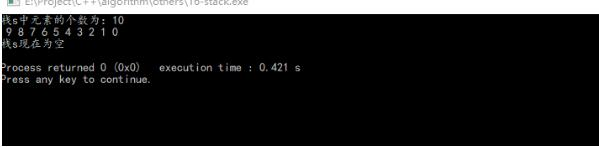# C++中stack、queue、vector的用法详解

更新时间：2017年08月30日 11:07:49   作者：致Great

`#include<stack>`

• empty() 堆栈为空则返回真
• pop() 移除栈顶元素
• push() 在栈顶增加元素
• size() 返回栈中元素数目
• top() 返回栈顶元素

3.实例代码

```#include<iostream>
#include<stack>
using namespace std;
int main(){
//创建栈 s
stack<int> s;
//将元素压入栈
for(int i=0;i<10;i++){
s.push(i);
}
//  if(!s.empty()){
//    cout<<"栈s不是空的"<<endl;
//  }
cout<<"栈s中元素的个数为："<<s.size()<<endl;
while(!s.empty()){
cout<<" "<<s.top();//获取栈顶元素
s.pop();//弹出栈顶元素
}
cout<<endl;
if(s.empty()){
cout<<"栈s现在为空"<<endl;
}
return 0;
}````#include<queue>`

• empty() 判断队列是否为空，返回类型为bool
• size() 返回队列中元素的个数
• front() 返回队列队首元素
• back() 返回队列队尾元素
• push(ele) 将元素ele插入到队尾
• pop 队首元素出队

```#include<iostream>
#include<queue>
using namespace std;
int main(){
int ele,num,last;
queue<int> q;
for(int i=0;i<10;i++){
q.push(i);
}
if(!q.empty()){
cout<<"队列不是空的"<<endl;
}
num=q.size();
cout<<"队列q的长度为："<<num<<endl;
last=q.back();
cout<<"队列q最后一个元素："<<last<<endl;
for(int j=0;j<q.size();j++){
ele=q.front();
cout<<ele<<" ";
q.pop();
}
cout<<endl;
if(q.empty()){
cout<<"队列是空的"<<endl;
}
return 0;
}````#include<vector>`

• c.clear() 移除容器中所有数据。
• c.empty() 判断容器是否为空。
• c.erase(pos) 删除pos位置的数据
• c.erase(beg,end) 删除[beg,end)区间的数据
• c.front() 传回第一个数据。
• c.insert(pos,elem) 在pos位置插入一个elem拷贝
• c.pop_back() 删除最后一个数据。
• c.push_back(elem) 在尾部加入一个数据。
• c.resize(num) 重新设置该容器的大小
• c.size() 回容器中实际数据的个数。
• c.begin() 返回指向容器第一个元素的迭代器
• c.end() 返回指向容器最后一个元素的迭代器

```#include<iostream>
#include<vector>
using namespace std;
int main(){
//向向量v中添加元素
vector<int> v;
for(int i=0;i<10;i++){
v.push_back(i);
}
//从向量v中读取数据
for(int i=0;i<v.size();i++){
cout<<" "<<v[i];
}
cout<<endl;
//使用迭代器遍历数据 迭代器相当于指针
cout<<"使用迭代器获取数据"<<endl;
vector<int>::iterator iter;
for( iter = v.begin(); iter != v.end(); iter++ )
{
cout<<" "<< *iter;
}
cout<<endl;
//删除数据
for(vector<int>::iterator it=v.begin();it!=v.end();){
v.erase(it);
}
if(v.empty()){
cout<<"向量v为空"<<endl;
}
return 0;
}```